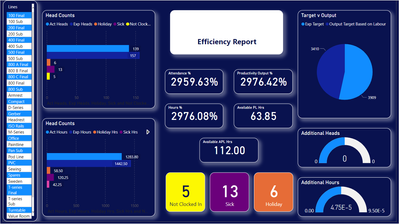cancel
Showing results for
Did you mean:Anonymous
Not applicable

## How to do a calculation in the total row

Hi

Totally new to BI so everything is new to me. I have this report that I have created:The 4 boxes in the centre below the white box display the totals for each column, which the system generates automatically.
Is there a way to display an Average figure automatically instead of a sum? So it adds them all up and then divide by 33 (that's the number of lines I'm reporting on). I.e. the first box is displaying 2959.63%, divide it by 33 and this will give me 89.69% which is the figure that I require to be displayed.

As I say I am new and a bit green.

1 ACCEPTED SOLUTIONSolution Sage

Simple answer: Change the Aggregation in the Visual Setting from Sum to Average.

BUT, If the number you are aggregating is already a percentage, then it is usually NOT a good idea to show the "Average of the Percentage." Suppose you sell 500,000 of widget A at \$1 each, and that average is \$1. but you also sold 1 of widget B at \$10. Your data may indicate that you have TWO averages, one per Widget type: \$1 and \$10. The Average of those two averages is \$5.50 (\$11 divided by 2). But the TRUE average is 500,010 divided by 500,001. To calculate that, you need the SUM of the Sales and the COUNT of Sales, and create a MEASURE that does that math with a DIVIDE statement.

Si

3 REPLIES 3Anonymous
Not applicable

Thanks for the response - Yes I know the math it's just how I apply that in to BI. Which, if I'm reading you right, is by creating a measure. Cool, I appreciate the direction.Solution Sage

Yes, a simple MEASURE.

And by the way, that MEASURE calculation will still exist at every sub-slice of data in that table, so if you slice/filter it by Product, or Date, or Customer, the calculation will only act on the rows of the slice, right down to a single row.

You actually have to work hard to make your measure calculations look at something other than the current slice/context.

Please mark replie as Solved if appropriate.

ThanksSolution Sage

Simple answer: Change the Aggregation in the Visual Setting from Sum to Average.

BUT, If the number you are aggregating is already a percentage, then it is usually NOT a good idea to show the "Average of the Percentage." Suppose you sell 500,000 of widget A at \$1 each, and that average is \$1. but you also sold 1 of widget B at \$10. Your data may indicate that you have TWO averages, one per Widget type: \$1 and \$10. The Average of those two averages is \$5.50 (\$11 divided by 2). But the TRUE average is 500,010 divided by 500,001. To calculate that, you need the SUM of the Sales and the COUNT of Sales, and create a MEASURE that does that math with a DIVIDE statement.

SiAnnouncements#### Exclusive opportunity for Women!

Join us for a free, hands-on Microsoft workshop led by women trainers for women where you will learn how to build a Dashboard in a Day!#### Power Platform Conference-Power BI and Fabric Sessions

Join us Oct 1 - 6 in Las Vegas for the Microsoft Power Platform Conference.Top Solution Authors
Top Kudoed Authors
Users online (3,120)